Courses

# Pumping Lemma for Regular Languages Computer Science Engineering (CSE) Notes | EduRev

## Computer Science Engineering (CSE) : Pumping Lemma for Regular Languages Computer Science Engineering (CSE) Notes | EduRev

The document Pumping Lemma for Regular Languages Computer Science Engineering (CSE) Notes | EduRev is a part of the Computer Science Engineering (CSE) Course Theory of Computation.
All you need of Computer Science Engineering (CSE) at this link: Computer Science Engineering (CSE)

Part VIII. Pumping Lemma for Regular Languages

Regular Languages
Regular languages are those classes of languages accepted by DFA’s and by NFAs. These languages can be defined
using regular expressions.
Not every language is regular.
For example,
Language defined by, L = {anbn for n = 0, 1, 2, 3, ....} is not regular.
Language defined by, L = {anban for n = 0, 1, 2, 3, ....} is not regular.
Language defined by, L = {anbnaabn+1 for n = 1, 2, 3, ....} is not regular.

A powerful technique, known as Pumping Lemma can be used to show that certain languages are not regular.

Pumping Lemma for Regular Languages

Definition
Let L be a regular language. Then, there exists a constant n such that
for every string w in L and |w| ≥ n,
w can be broken into three parts, x , y and z such that
w = xyz, y /∈ ε, and |xy| ≤ n.
Then for all i ≥ 0, the string xyiz also belongs to L.

That is, we always find a non-empty string y not too far from the beginning of w that can be “pumped”; that is, repeating y any number of times, keeps the resulting string in the language.

A Simple Definition
Let L be a language and w be a string in the language. Break w into three parts. Then write, w = xyz.
Then xyiz must also be a string in the language. That is, strings xy0z, xy1z , xy2z , xy3z , xy4z ...... must be in the language.
Then L is a context free language.
For example,

Example 1:
Consider the language L = {an|n ≥ 1}. This language is regular.

The set of strings in this language are,
{a, aa, aaa, aaaa, aaaaa, .........}

Consider one string in this language, aaaa.
Let w=aaaa.
Break w into three parts, x, y and z.
Let w=aaaa=xyz
That is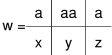Then,
xyiz is
Let i=1,
we have, xy1z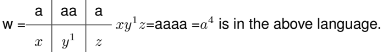Let i=2,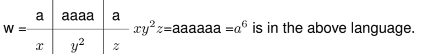Let i=3,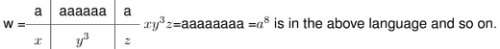Since all the strings of the form xyiz , are in the above language, the language an is a regular language.

Example 2:
Consider the language L = {anbm|n, m ≥ 1}. This language is regular.

The set of strings in this language are,
{ab, abb, abbb, aab, aabb,aaaabbbbbbbb .........}
Consider one string in this language, aaabb.
Let w=aaabb.
Break w into three parts, x, y and z.
Let w=aaabb=xyz
That is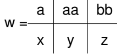Then,
xyiz is
Let i=1,
we have, xy1z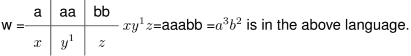Let i=2,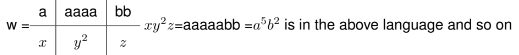Since all the strings of the form xyiz , are in the above language, the language anbm is regular.

Example 3:
Consider the language L = {anbn|n ≥ 1}. This language is not regular.

The set of strings in this language are,
{ab, aabb, aaabbb, aaaabbbb, aaaaabbbbb, .........}
Consider one string in this language, aaabbb.
Let w=aaabbb.
Break w into three parts, x, y and z.
Let w=aaabbb=xyz
That is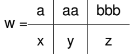Then,
xyiz is
Let i=1,
we have, xy1z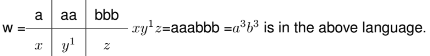Let i=2,
we have, xy2z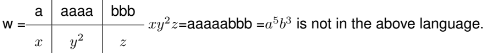Since some of the strings of the form xyiz , are not in the above language, the language anbn is not regular.

Proof

Let L is regular. Then a DFA exists for L.
Let that DFA has n states.
Consider a string w of length n or more, let w = a1, a2, a3.....am, where m≥n and each ai is an input symbol
By the Pigeonhole principle, it is not possible for the n+1 different states (Pi’s) for i=0,1,2,3....n to be distinct, since there are only n states.
Thus we can find two different integers i and j such that Pi = Pj .
Now we can break w = xyz as follows:
1. x = a1a2, .......ai
2. y = ai+1ai+2.......aj
3. z = aj+1aj+2......am

That is x takes us to Pi once,
y takes us from Pi back to Pi (since Pi is also Pj ), and z is balance of w.
This is shown in the following diagram.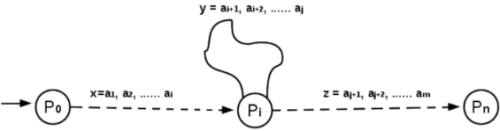Note that x may be empty, when i=0.
Also, z may be empty, if j = n = m.
y cannot be empty, since i is strictly less than j.

Suppose the above automation receives xyiz for an i ≥ 0.
If i = 0, then the string is xz,
automation goes from the start state P0 to Pon input x.
Since Pis also Pj , it must be that M goes from Pi to the accepting state on input z.
Thus xz is accepted by the automation.
That is,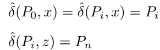If i>0, then
automation goes from P0 to Pon input string x,
circles from Pi to Pi, i times on input yand then goes to the accepting state on input z.
Thus xyiz is accepted by the automation.
That is,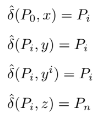Pumping lemma is useful for proving that certain languages are not regular.

Proving Non-regularity of certain Languages using Pumping Lemma
To prove that certain languages are not regular, following are the steps:
Step 1:
Assume that L is regular. Let n be the number of steps in the corresponding finite automation.
Step 2:
Choose a string w such that |w| ≥ n. Use pumping lemma to write w = xyz, with |xy| ≤ n and |y| > 0.
Step 3:
Find a suitable integer i such that xyiz ∉ L. This contradicts our assumption. Hence L is not regular.

The important part of proof is Step 3. There, we need to find i such that xyiz ∉ L.
In some cases, we prove xyiz ∉ L by considering |xyiz|.
In some cases, we may have to use the structure of strings in L.

Example 1:
Prove that language, L = {0i1for i ≥ 1 } is not regular.

Step 1: Assume that L is regular. Let n be the number of steps in the corresponding finite automation.
Step 2: Choose a string w such that |w| ≥ n. Use pumping lemma to write w = xyz, with |xy| ≤ n and |y| > 0.

Let w = 0n1n.
Then |w| = 2n > n.
By pumping lemma, we can write w = xyz, with |xy| ≤ n and |y| 6 ≠ 0.

Step 3: Find a suitable integer i such that xyiz ∉ L. This contradicts our assumption. Hence L is not regular.

The string y can be any one of the following forms:
Case 1: y has only 0’s, ie. y = 0k for some k ≥ 1.
In this case, we can take i=0. As xyz = 0n1n, xz = 0nk1n.

As k ≥ 1, n − k 6= n. So, xz /∈ L.
Case 2: y has only 1’s, ie. y = 1m, for some m ≥ 1.
In this case, take i=0.
As before, xz is 0n1n−m and n 6 ≠ n − m. So, xz / ∉ L.

Case 3: y has both 0’s and 1’s. ie. y = 0k1j, for some k, j ≥ 1
In this case, take i=2.
As xyz = 0n−k0k1j1n−j,xy2z = 0n−k0k1j0k1j1n−j,
As xy2z is not of the form 0i1i, xy2z ∉ L.
In all three cases, we get a contradiction. So L is not regular.

Example 2:
Prove that L = {ai2|i ≥ 1} is not regular.

Proof:

Step 1: Assume that L is regular. Let n be the number of steps in the corresponding finite automation.
Step 2: Choose a string w such that |w| ≥ n. Use pumping lemma to write w = xyz, with |xy| ≤ n and |y| > 0.
Let w = an2. Then |w| = n2 > n.
By pumping lemma, we can write w = xyz with |xy| ≤ n and |y| > 0.

Step 3: Find a suitable integer i such that xyiz /∈ L. This contradicts our assumption. Hence L is not regular.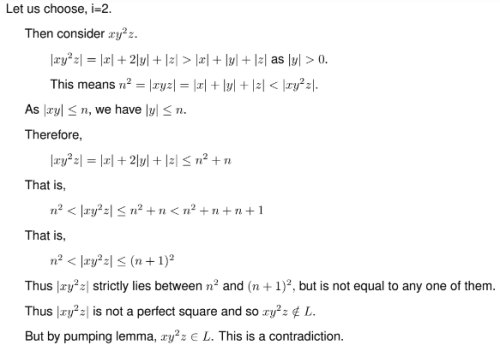Example 3:
Prove that L = {ap|p is a prime} is not regular.

Proof:

Step 1: Assume that L is regular. Let n be the number of steps in the corresponding finite automation.
Step 2: Choose a string w such that |w| ≥ n. Use pumping lemma to write w = xyz, with |xy| ≤ n and |y| > 0.

Let p be a prime number greater than n.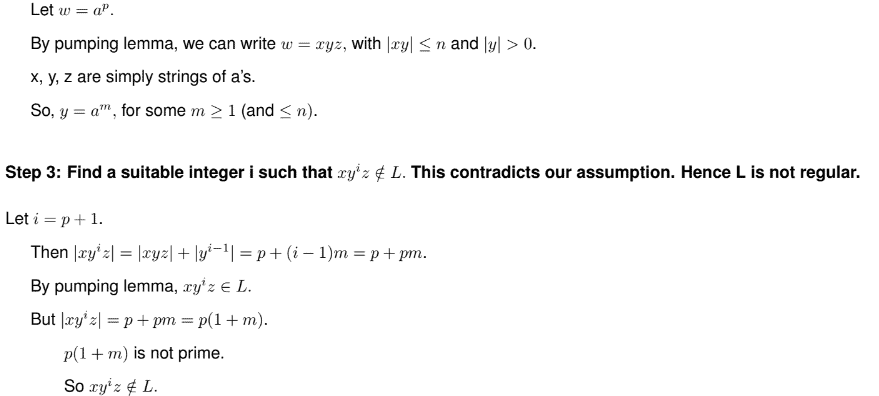This is a contradiction.
Thus L is not regular.

Example 4:
Show that L = {ww|w ∈ {a, b}*} is not regular.

Proof:

Step 1: Assume that L is regular. Let n be the number of steps in the corresponding finite automation.
Step 2: Choose a string w such that |w| ≥ n. Use pumping lemma to write w = xyz, with |xy| ≤ n and |y| > 0.

Let us consider ww = anbanb in L.
|ww| = 2(n + 1) > n
We can apply pumping lemma to write
ww = xyz with |y| 6 ≠ 0, |xy| ≤ n.

Step 3: Find a suitable integer i such that xyiz ∉ L. This contradicts our assumption. Hence L is not regular.

We want to find i so that xyiz ∉ L for getting a contradiction.
The string y can be in only one of the following forms:
Case 1: y has no b’s. ie. y = afor some k ≥ 1.

Case 2: y has only one b.
We may note that y cannot have two b’s. If so, |y| ≥ n + 2. But |y| ≤ |xy| ≤ n.
In case 1, we can take i = 0. Then xy0z = xz is of the form ambanb, where m = n − k < n. We cannot write xz in the form uu with u ∈ {a, b}*, and
so xz ∉ L.

In case 2 also, we can take i = 0. Then xy0z = xz has only one b ( as one b is removed from xyz, b being in y). So xz ∉ L as any element in L should have an even number of a’s and an even number of b’s. Thus in both cases, we have a contradiction. So, L is not regular.

Offer running on EduRev: Apply code STAYHOME200 to get INR 200 off on our premium plan EduRev Infinity!

## Theory of Computation

18 videos|43 docs|39 tests

,

,

,

,

,

,

,

,

,

,

,

,

,

,

,

,

,

,

,

,

,

;